# Part 8: Pythagoras’ Theorem | Free Worksheet

Pythagoras' theorem might seem a little intimidating at first. But it doesn't have to be! In this article, we will investigate how Pythagoras' theorem works to develop your understanding, and provide you worked examples to help you apply this theorem for Year 7 Maths.The concept of Pythagoras’ Theorem is of great importance, pivotal to numerous areas of mathematics and science study.

A solid understanding of the theorem allows the student to determine unknown sides and angles in right-angled triangles.

As such, it has many real-world applications like window construction to ensure accurate dimensions or calculating the length of any diagonals (eg. the screen size of a television).

## NSW Syllabus Outcome

 Syllabus Explanation NSW Stage 4 NESA Syllabus Investigate Pythagoras’ theorem and its application to solving simple problems involving right-angled triangles This means that you understand how Pythagoras’ theorem relies on squared numbers ($$x^2$$ and its relationship with right-angled triangles Use Pythagoras’ theorem to find the length of an unknown side in a right-angled triangle This means that you can solve questions like “Find the length of the hypotenuse for the following triangle.”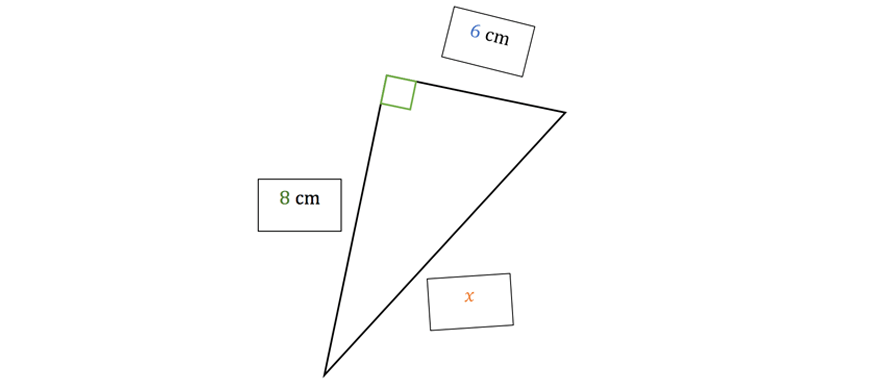Apply Pythagoras’ theorem to solve problems involving the perimeters and areas of plane shapes (Problem Solving) This means that you can solve questions like “Find the perimeter of the above triangle, using Pythagoras’ theorem“ Use the converse of Pythagoras’ theorem to establish whether a triangle has a right angle This means that you can see if a triangle has a right angle by seeing if all the sides satisfy Pythagoras’ theorem.

## Test your knowledge of Pythagoras Theorem## Investigation

The discovery of new mathematical ideas often emerges from investigating patterns in old ones.

They can be extremely interesting when you think about why they would be true.

Let’s see an example now.

Example

Recall. Remember we have invented a useful notation when we want to multiply the same number with itself over and over again – indices.

Eg. We can write $$\underbrace{3 \times 3 \times 3 \times 3}_{\color{blue}{four \ copies}} = 3^{\color{blue}{4}}$$.

The number of things that we have becomes the power.

Recall. We will be particularly interested in square numbers in this subject guide.

A square number always has a power of two.

Eg. $$5 \times 5$$ means $$25$$.

1. Write down what the value of the following square numbers is.

 $$0^{2} = ?$$ $$8^{2} =?$$ $$1^{2} = ?$$ $$12^2 = ?$$ $$2^{2} = ?$$ $$13^2 =?$$ $$3^{2} = ?$$ $$15^2 = ?$$ $$4^{2} = ?$$ $$17^{2} =?$$ $$5^{2} = ?$$ $$24^{2} = ?$$

A strange pattern.

Now we know what square numbers are. But have you noticed this before:

\begin{align*}
3^{2} + 4^{2} = 5^{2}?
\end{align*}

\begin{align*}
5^{2} + 12^{2} = 13^{2}?
\end{align*}

2. Try to figure out what number $$c$$ is if

\begin{align*}
7^{2} + 24^{2} = c^{2}?
\end{align*}

Questions arise.

Hopefully you found that $$c = 25$$ in the question above.

But some natural questions arise:

• Why did those equations work?
• Why don’t they always work?

For example, if you tried

\begin{align*}
1^{2} + 2^{2} ≠ 3^{2}
\end{align*}

In fact, $$1^{2} + 2^{2} = 5$$ which is not even a square number at all?

And finally, could there be any practical usefulness of this?

One final investigation.

3. You will need a ruler, grid paper, and a pencil.

On a piece of grid paper, draw up the right-angled triangle below. Make sure the side lengths match the ones in the picture below!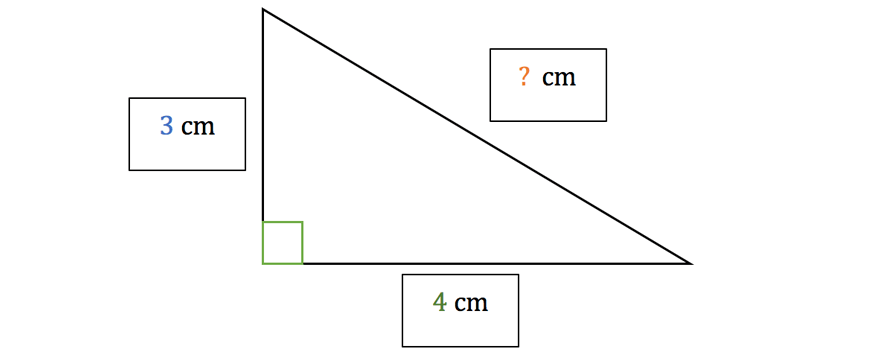Then, carefully measure the length of the “longest side” – the side with unknown length.

You should get something which is approximately $$\color{orange}{5} cm$$.

That’s a little strange?

Because from before $$\color{blue}{3}^{2} + \color{green}{4}^{2} = \color{orange}{5}^{2}$$.

4. Try drawing another right-angled triangle, this time with side lengths $$5cm$$ (instead of $$3cm$$ ) and $$12cm$$  (instead of $$4cm$$ ). What is the length of the “longest side” this time?

Which equation does this remind us of this time?

A Greek philosopher and mathematician more than $$2500$$ years ago was coincidently wondering the same thing.

He was the first one to prove a special relationship between square numbers and right-angled triangles.

His name was Pythagoras, and finally, here is the answer:

### Pythagoras’ theorem.

Firstly, lets introduce a new word.

We mentioned “the longest side” of a right-angled triangle multiple times – and this takes a long time to say.

The standard word used to describe this is the hypotenuse.

It is the longest side of a right-angled triangle and it is always opposite the right angle.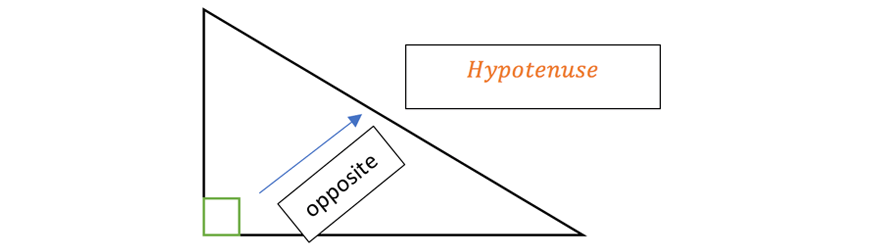For convenience, and to make our diagram look a little less cluttered, lets stick with the following: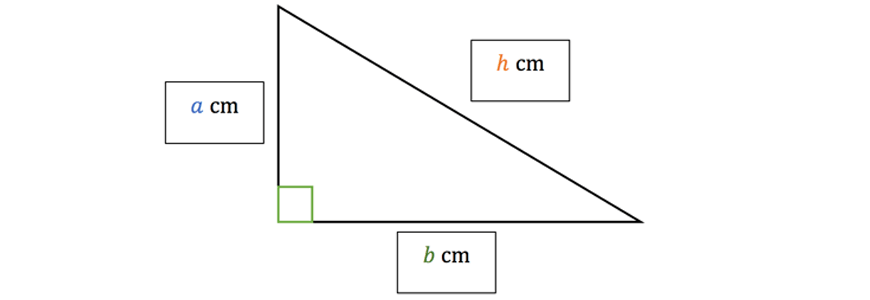Pythagoras’ theorem: If you square the length of the hypotenuse, you will get the sum of the squares of the length of the other two sides. $$\color{blue}{a}^{2} + \color{green}{b}^{2} = \color{orange}{h}^{2}$$

Note: You will see this in the example questions, but the letters that we label the sides of the triangle might change from question to question.

But Pythagoras’ theorem always stays as the same formula.

That way you should always think about it is:

\begin{align*}
\color{green}{\underbrace{(shorter \ side)^2}_{b^2}} \color{red}{+} \color{blue}{\underbrace{(other \ shorter \ side)^2}_{a^2}} \color{red}{=} \color{orange}{\underbrace{(hypotenuse)^2}_{h^2}}
\end{align*}

Q) Why did those equations work? (E.g. $$3^{2} + 4^{2} = 5^{2}$$ )

A) Consider a triangle with shorter side lengths being $$3$$ and $$4$$.

By Pythagoras’ theorem, this must equal to the square of the hypotenuse length.

From our investigation above, we found the hypotenuse of that triangle was $$5$$.

This means that $$3^{2} + 4^{2} = 5^{2}$$.

A) Anytime you are adding square numbers (e.g. $$5^{2} +12^2$$), you can think of this as using Pythagoras’ theorem on a triangle with side lengths $$5$$ and $$12$$.

Depending on the length of the hypotenuse, the sum will be $$h^2$$.

In the case of a triangle with smaller side lengths $$5$$ and $$12$$, the hypotenuse has length $$13$$.

Hence, we will get $$5^{2} +12^2 = 13^2$$.

Q) Why don’t they always work? (e.g. $$1^2 + 2^{2} ≠ 3^{2}$$)

A) The examples we saw were “coincidently” nice.

However, the example where we did $$1^2 + 2^{2} = 5$$, the length of the hypotenuse would actually be $$\sqrt{5}$$.

You only get a whole number squared of the right when you have a right-angled triangle where the side lengths are all whole numbers.

The cases where we have nice equations (and are all whole numbers) are called Pythagorean triads.

You will end up memorising a bunch of these when you use the formula in the example questions!

Q) Could there be any practical applications for this?

A) Yes!

The way we “discovered” Pythagoras’ theorem, by thinking about patterns in square numbers, is actually a very small part of the theorem.

If you think about it, if $$a^2 + b^{2}$$ always equals $$h^{2}$$…

This means whenever you have two sides of a right-angled triangle, you can always use Pythagoras’ theorem to find the length of the third side.

This is incredibly useful!

It was probably a little tedious when you had to draw up those triangles and measure the sides carefully in our investigation.

And even worse – we would be prone to all sorts of measurement errors!

Pythagoras’ Theorem lets us calculate what the exact length of the third side will be – without any errors, given you already have two sides.

## Using Pythagoras’ theorem – Worked Examples

Now, we will focus on what you need to know about Pythagoras’ Theorem for school.

We will go through some standard examples you will see in tests.

We strongly recommend that you try the questions yourself before looking at the solutions! (It’s always more fun figuring out the question yourself!)

Pythagoras’ Theorem is almost always used to find sides in a right-angled triangle.

Example

1. Find the length of the hypotenuse for the following triangle.Solution:

Recall the hypotenuse is the longest side of a right-angled triangle and it is always opposite the right angle.

In our case, the length of hypotenuse is given by $$x$$.

We know by Pythagoras’ theorem that:

\begin{align*}
\color{green}{(shorter \ side)^2} + \color{blue}{(other \ shorter \ side)^{2}} &= \color{orange}{(hypotenuse)^{2}} \\
8^{2} + 6^{2} &= x^{2} \\
64 + 36 &= x^{2} \\
x^{2} &= 100 \\
x &= \sqrt{100} \\
x &= 10
\end{align*}

2. Find an expression for $$x$$ in terms of  $$m$$ and  $$n$$ using Pythagoras’ theorem.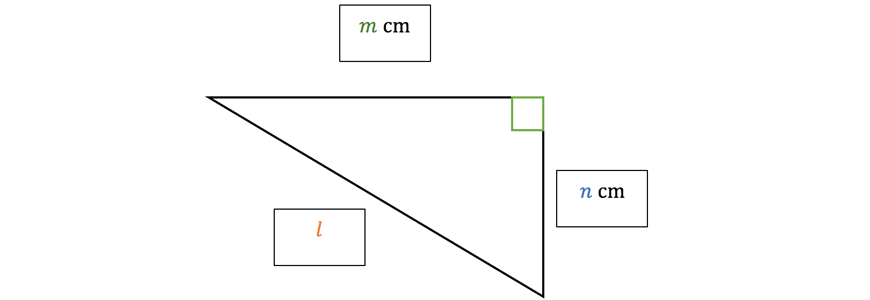Solution:

Firstly, lets recall what “an expression for $$l$$ in terms of $$m$$ and $$n$$” means.

That means

Write $$l =$$ stuff with $$m$$ and $$n$$

That is, we need to write down a relationship between  $$l$$ and $$m/n$$.

Let’s use Pythagoras’ theorem to do this.

\begin{align*}
\color{green}{(shorter \ side)^{2}} + \color{blue}{(other \ shorter \ side)^{2}} &= \color{orange}{(hypotenuse)^{2}} \\
m^2 + n^2 &= l \\
l^{2} &= m^2 + n^{2} \\
l &= \sqrt{m^{2} + n^{2}}
\end{align*}

And that’s our “expression”!

3. Find the value of $$y$$ for the following triangle.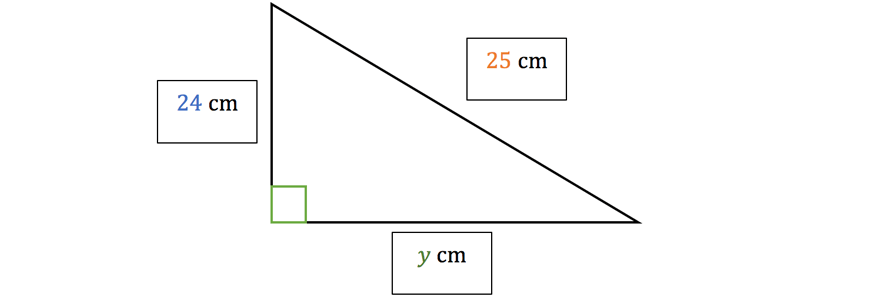Solution:

We use Pythagoras’ theorem to do this.

Notice this time, the side length we are trying to find is not the hypotenuse.

The hypotenuse is already given to be $$25cm$$.

This time, we are finding the length of a shorter side.

Fortunately, we can still use our formula and rearrange to figure out what $$y$$ is!

\begin{align*}
\color{green}{(shorter \ side)^{2}} + \color{blue}{(other \ shorter \ side)^{2}} &= \color{orange}{(hypotenuse)^2} \\
y^{2} + 24^{2} &= 25^2 \\
y^2 &= 25^{2} – 24^{2} \\
y^2 &= 49 \\
y^2 &= 7
\end{align*}

Hence the length of the unknown side is $$7cm$$.

4. Find the exact length of the diagonal $$AC$$ if the square $$ABCD$$ below has a side length of $$8cm$$ cm.

(Hint, draw the diagonal and spot a right-angled triangle!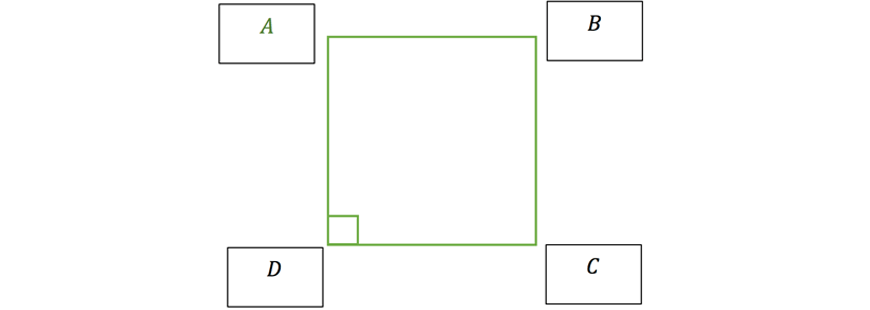Solution:

This question is a little tricker for two reasons.

• There’s no triangle?
• There’s no visible “missing length?

But actually the question is asking for the length of the diagonal $$AC$$.

So like the hint says, (but this should make sense to do), we should draw in the line $$AC$$ and see what happens.

While we do that, lets label the side length as $$8cm$$ since the question tells us this too.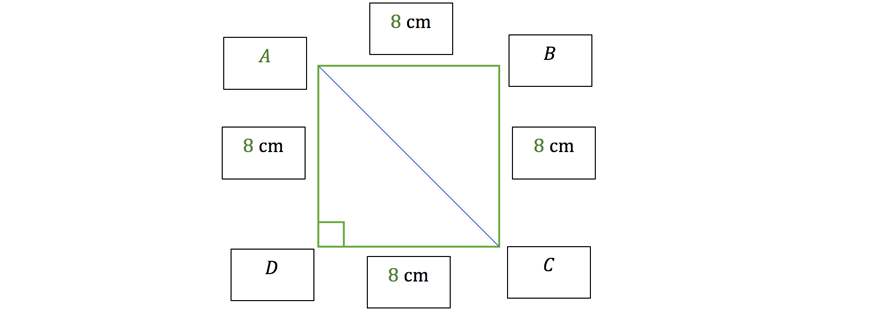Now we have a right-angled triangle $$ACD$$!

We can use Pythagoras’ theorem to figure out the length of $$AC$$.

Notice that there’s no “letter” like $$x$$ or $$y$$ attached to the side $$AC$$.

So, you can either put a letter there yourself, or just use $$AC$$ as the variable.

\begin{align*}
\color{green}{(shorter \ side)^{2}} + \color{blue}{(other \ shorter \ side)^{2}} &= \color{orange}{(hypotenuse)^{2}} \\
8^{2} + 8^{2} &= AC^{2} \\
64 + 64 &= AC^2 \\
AC^2 &= 128 \\
AC &= \sqrt{128} \\
&= 16\sqrt{2}
\end{align*}

We are going to leave our answer like this, instead of popping it in the calculator.

This is because the question asked for an exact length.

Whenever the question asks this, you always leave you answer as a simplified square root.

If you put it in your calculator, you will get a never-ending decimal, and you will need to round your answer.

This “rounding” means the number you wrote down wasn’t $$100%$$ correct, since we left a few numbers out.

This question actually illustrates a few important and interesting things.

1. Not only is Pythagoras’ theorem useful for right-angled triangles, it can be very useful for other shapes which have right-angled triangles hidden inside of them as well!

2. The question will not always give us the side to find, and letters/variables labelled on the diagram for us to plug into the formula.

But all you need to do is label them yourself!

Remember labelling sides of a triangle with letters is just saving yourself from having to write out a long formula like:

\begin{align*}
\color{green}{(shorter \ side)^{2}} + \color{blue}{(other \ shorter \ side)^{2}} &= \color{orange}{(hypotenuse)^{2}}
\end{align*}

Instead, we can just write something like:

\begin{align*}
a^2 + b^2 = h^2
\end{align*}

depending on how you names the sides of your triangle

## Need more practice with Pythagoras’ Theorem?

At Matrix, our comprehensive resource booklets will help you get the practice you need! Our countless of practice questions and clear explanations of key concepts will help boost your marks.